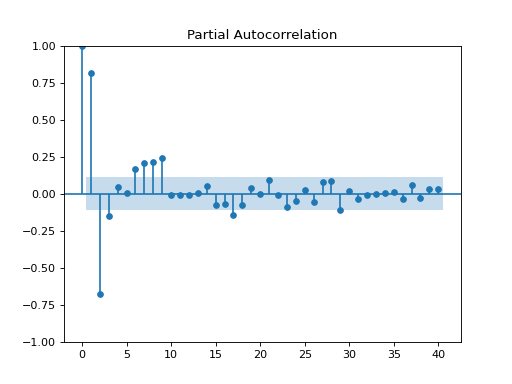# statsmodels.graphics.tsaplots.plot_pacf¶

`statsmodels.graphics.tsaplots.``plot_pacf`(x, ax=None, lags=None, alpha=0.05, method='ywadjusted', use_vlines=True, title='Partial Autocorrelation', zero=True, vlines_kwargs=None, **kwargs)[source]

Plot the partial autocorrelation function

Parameters
xarray_like

Array of time-series values

ax`AxesSubplot`, `optional`

If given, this subplot is used to plot in instead of a new figure being created.

lags{`int`, array_like}, `optional`

An int or array of lag values, used on horizontal axis. Uses np.arange(lags) when lags is an int. If not provided, `lags=np.arange(len(corr))` is used.

alpha`float`, `optional`

If a number is given, the confidence intervals for the given level are returned. For instance if alpha=.05, 95 % confidence intervals are returned where the standard deviation is computed according to 1/sqrt(len(x))

method{‘ywunbiased’, ‘ywmle’, ‘ols’}

Specifies which method for the calculations to use:

• yw or ywunbiased : yule walker with bias correction in denominator for acovf. Default.

• ywm or ywmle : yule walker without bias correction

• ols - regression of time series on lags of it and on constant

• ld or ldunbiased : Levinson-Durbin recursion with bias correction

• ldb or ldbiased : Levinson-Durbin recursion without bias correction

use_vlinesbool, `optional`

If True, vertical lines and markers are plotted. If False, only markers are plotted. The default marker is ‘o’; it can be overridden with a `marker` kwarg.

title`str`, `optional`

Title to place on plot. Default is ‘Partial Autocorrelation’

zerobool, `optional`

Flag indicating whether to include the 0-lag autocorrelation. Default is True.

vlines_kwargs`dict`, `optional`

Optional dictionary of keyword arguments that are passed to vlines.

**kwargs`kwargs`, `optional`

Optional keyword arguments that are directly passed on to the Matplotlib `plot` and `axhline` functions.

Returns
`Figure`

If ax is None, the created figure. Otherwise the figure to which ax is connected.

Notes

Plots lags on the horizontal and the correlations on vertical axis. Adapted from matplotlib’s xcorr.

Data are plotted as `plot(lags, corr, **kwargs)`

kwargs is used to pass matplotlib optional arguments to both the line tracing the autocorrelations and for the horizontal line at 0. These options must be valid for a Line2D object.

vlines_kwargs is used to pass additional optional arguments to the vertical lines connecting each autocorrelation to the axis. These options must be valid for a LineCollection object.

Examples

```>>> import pandas as pd
>>> import matplotlib.pyplot as plt
>>> import statsmodels.api as sm
```
```>>> dta = sm.datasets.sunspots.load_pandas().data
>>> dta.index = pd.Index(sm.tsa.datetools.dates_from_range('1700', '2008'))
>>> del dta["YEAR"]
>>> sm.graphics.tsa.plot_acf(dta.values.squeeze(), lags=40)
>>> plt.show()
```